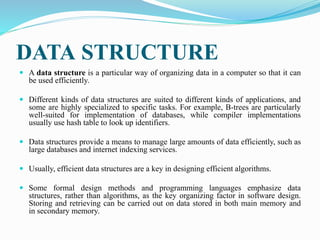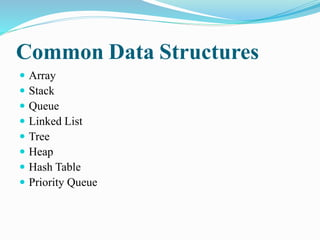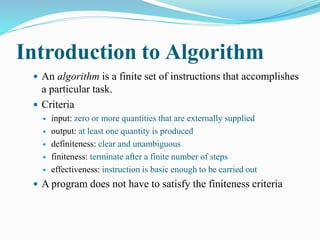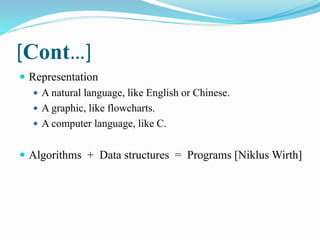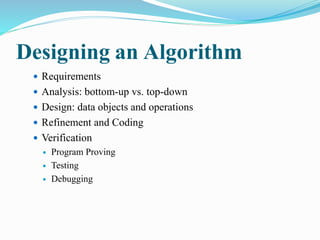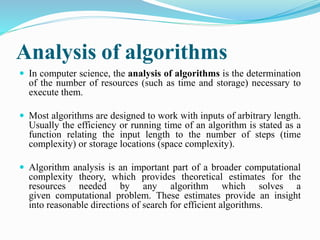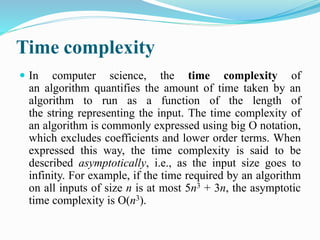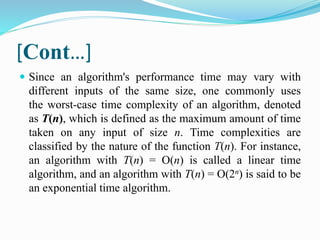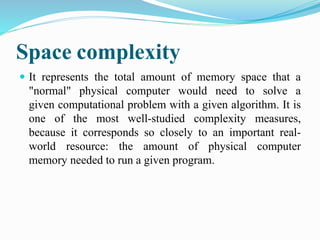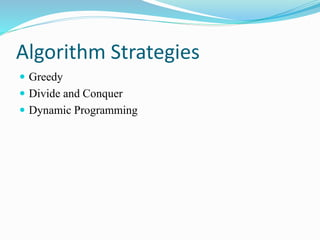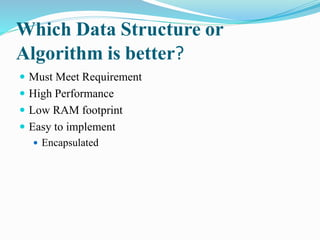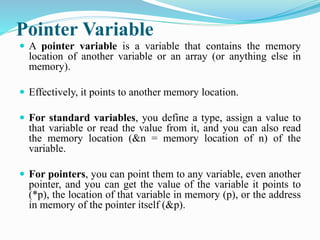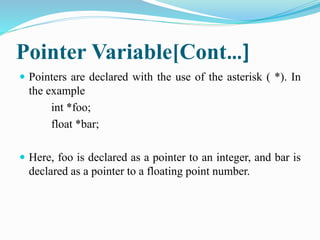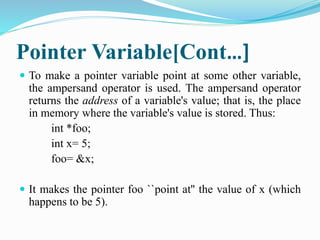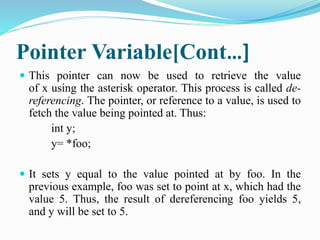1 de 18

### Data structure and algorithm

1. What is Program  A Set of Instructions  Data Structures + Algorithms  Data Structure = A Container stores Data  Algorithm = Logic + Control Trupti Agrawal 1
2. DATA STRUCTURE  A data structure is a particular way of organizing data in a computer so that it can be used efficiently.  Different kinds of data structures are suited to different kinds of applications, and some are highly specialized to specific tasks. For example, B-trees are particularly well-suited for implementation of databases, while compiler implementations usually use hash table to look up identifiers.  Data structures provide a means to manage large amounts of data efficiently, such as large databases and internet indexing services.  Usually, efficient data structures are a key in designing efficient algorithms.  Some formal design methods and programming languages emphasize data structures, rather than algorithms, as the key organizing factor in software design. Storing and retrieving can be carried out on data stored in both main memory and in secondary memory.
3. Common Data Structures  Array  Stack  Queue  Linked List  Tree  Heap  Hash Table  Priority Queue
4. Introduction to Algorithm  An algorithm is a finite set of instructions that accomplishes a particular task.  Criteria  input: zero or more quantities that are externally supplied  output: at least one quantity is produced  definiteness: clear and unambiguous  finiteness: terminate after a finite number of steps  effectiveness: instruction is basic enough to be carried out  A program does not have to satisfy the finiteness criteria
5. [Cont…]  Representation  A natural language, like English or Chinese.  A graphic, like flowcharts.  A computer language, like C.  Algorithms + Data structures = Programs [NiklusWirth]
6. Designing an Algorithm  Requirements  Analysis: bottom-up vs. top-down  Design: data objects and operations  Refinement and Coding  Verification  Program Proving  Testing  Debugging
7. Approaches for Designing an Algorithm
8. Analysis of algorithms  In computer science, the analysis of algorithms is the determination of the number of resources (such as time and storage) necessary to execute them.  Most algorithms are designed to work with inputs of arbitrary length. Usually the efficiency or running time of an algorithm is stated as a function relating the input length to the number of steps (time complexity) or storage locations (space complexity).  Algorithm analysis is an important part of a broader computational complexity theory, which provides theoretical estimates for the resources needed by any algorithm which solves a given computational problem. These estimates provide an insight into reasonable directions of search for efficient algorithms.
9. Time complexity  In computer science, the time complexity of an algorithm quantifies the amount of time taken by an algorithm to run as a function of the length of the string representing the input. The time complexity of an algorithm is commonly expressed using big O notation, which excludes coefficients and lower order terms. When expressed this way, the time complexity is said to be described asymptotically, i.e., as the input size goes to infinity. For example, if the time required by an algorithm on all inputs of size n is at most 5n3 + 3n, the asymptotic time complexity is O(n3).
10. [Cont…]  Since an algorithm's performance time may vary with different inputs of the same size, one commonly uses the worst-case time complexity of an algorithm, denoted as T(n), which is defined as the maximum amount of time taken on any input of size n. Time complexities are classified by the nature of the function T(n). For instance, an algorithm with T(n) = O(n) is called a linear time algorithm, and an algorithm with T(n) = O(2n) is said to be an exponential time algorithm.
11. Space complexity  It represents the total amount of memory space that a "normal" physical computer would need to solve a given computational problem with a given algorithm. It is one of the most well-studied complexity measures, because it corresponds so closely to an important real-world resource: the amount of physical computer memory needed to run a given program.
12. Algorithm Strategies  Greedy  Divide and Conquer  Dynamic Programming
13. Which Data Structure or Algorithm is better?  Must Meet Requirement  High Performance  Low RAM footprint  Easy to implement  Encapsulated
14. Pointer Variable  A pointer variable is a variable that contains the memory location of another variable or an array (or anything else in memory).  Effectively, it points to another memory location.  For standard variables, you define a type, assign a value to that variable or read the value from it, and you can also read the memory location (&n = memory location of n) of the variable.  For pointers, you can point them to any variable, even another pointer, and you can get the value of the variable it points to (*p), the location of that variable in memory (p), or the address in memory of the pointer itself (&p).
15. Pointer Variable[Cont…]  Pointers are declared with the use of the asterisk ( *). In the example int *foo; float *bar;  Here, foo is declared as a pointer to an integer, and bar is declared as a pointer to a floating point number.
16. Pointer Variable[Cont…]  To make a pointer variable point at some other variable, the ampersand operator is used. The ampersand operator returns the address of a variable's value; that is, the place in memory where the variable's value is stored. Thus: int *foo; int x= 5; foo= &x;  It makes the pointer foo ``point at'' the value of x (which happens to be 5).
17. Pointer Variable[Cont…]  This pointer can now be used to retrieve the value of x using the asterisk operator. This process is called de-referencing. The pointer, or reference to a value, is used to fetch the value being pointed at. Thus: int y; y= *foo;  It sets y equal to the value pointed at by foo. In the previous example, foo was set to point at x, which had the value 5. Thus, the result of dereferencing foo yields 5, and y will be set to 5.
18. THANK YOU….. !!!